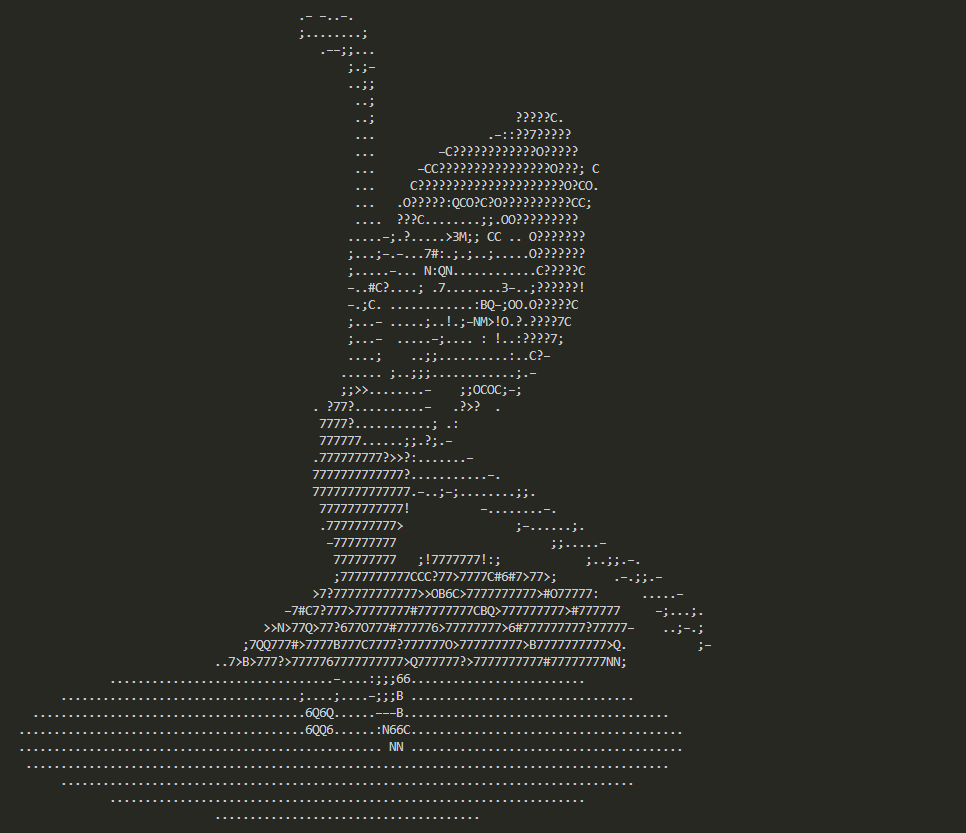# 怎么利用Python实现图片转字符画

### 灰度值和RGB

RGB 是红绿蓝三颜色，通过不同的叠加可得到其他颜色。

RGB 转灰度值，有个转换学公式：

gray = (2126 * r + 7152 * g + 722 * b) / 10000

### 代码实战```from PIL import Image
char = list('M3NB6Q#OC?7>!:–;. ')
def get_char(r, g, b, alpha=256):
if alpha == 0:
return ' '
grey = (2126 * r + 7152 * g + 722 * b) / 10000
char_idx = int((grey / (alpha + 1.0)) * len(char))
return char[char_idx]

def write_file(out_file_name, content):
with open(out_file_name, 'w') as f:
f.write(content)
def main(file_name="input.jpg", width=100, height=80, out_file_name='output.txt'):
text = ''
im = Image.open(file_name)
im = im.resize((width, height), Image.NEAREST)
for i in range(height):
for j in range(width):
text += get_char(*im.getpixel((j, i)))
text += '\n'
print(text)
write_file(out_file_name, text)

if __name__ == '__main__':
main('dance.png')```

• 定义一个字符数组，这个数组里面的字符可以随意写。

• 解析图片，将图片里面的每个像素点解析成 RGB 值。

• 根据我们上面的公式，将每个像素点转换成字符数组里面的一个字符。

• 将所有像素点对应的字符拼接起来，就完成了转换。